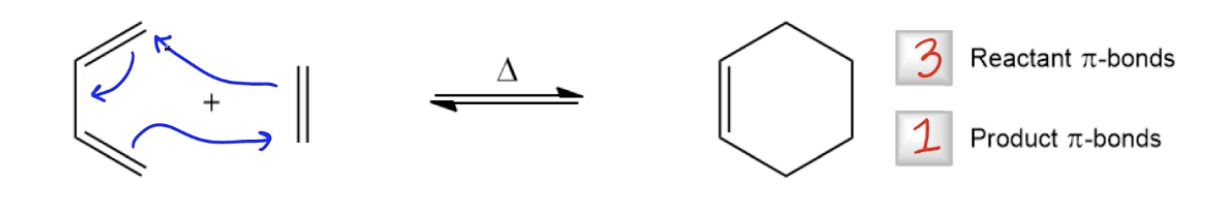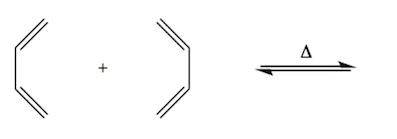Start typing, then use the up and down arrows to select an option from the list.# Organic Chemistry

Learn the toughest concepts covered in Organic Chemistry with step-by-step video tutorials and practice problems by world-class tutors.

16. Conjugated Systems

Thermal Cycloaddition reactions are pericyclic reactions in which 2 pi bonds are destroyed after a heat-activated cyclic mechanism.

1

#### concept13m
Play a video:2

#### example

Determining Favorability of HOMOb to LUMOa4m
Play a video:
Now, let's look at the favorability of going the other way around. Trying toe make a bonding interaction between Homo B and Lou Mo A. Okay, so this also means that we're gonna have to redraw our molecular orbital because they have changed. Homo B is pretty easy. We already drew this notice that it is this guy right here. So I'm just gonna bring that down home. A B would look like this quote now loom. Oh, hey, We didn't draw because limo is actually side three of the other molecule, so that means we have to draw it from scratch. Let's go ahead and draw it right here. One to three for so much room for improvement. That's not the best. So how do we draw this? Remember that for side three, this would continue to face down so it would be dark at the bottom. And remember that this gives my last orbital two chances to flip so it would have been down then on side to it flipped up and then on side three, it flips back down and then finally remember that I need how many nodes? Two notes because I need one increasing note with every M o. So that means that I would have a note here and a node here, meaning that I should have Orbital's the phases facing up in the middle. And that is my m o diagram for side three. This is what is interacting with homo be, and I'm gonna bring this down. Let's bring that down into this area. So what I have is down. Up, up, down. Awesome. Okay, so now we're ready to determine and to make some decisions about if this is gonna be favorable or non so. First of all, let's look at the symmetry of this reaction. Is this a symmetry allowed or a symmetry disallowed process. And guys, what I see is that actually, this is symmetry allowed again. Because what I have is that this one matches this one, right? So there could be a bond there, and then this one. Oops. I wanna use a different color. This terminal end matches this terminal end. Right? So, once again, the this is symmetry allowed bonds can form here. What I could do is I could form a bond here and a bond there. Cool. So we know that bonds could forms. This is symmetry allowed. Let's go to the next one. Is this the smallest Homo Lamo Gap possible? And what we see here is that I actually went ahead and I brought down the same exact distances from my original L CEO diagram into here. So notice that this is purposeful. The reason that the Homo and the loom Oh, look a little bit further apart in this one than they do on the first one is because they are further apart. If you actually look at them, they are drawn much further apart. And that's the way that it works. Usually there's gonna be one set that's closer. And once that that's further away. The set that's further away isn't gonna work as well. So this would get a big X in smallest home, a limo gap. Is it possible to make this one work? Yes, but then you would need to try to decrease, try to do different things to the molecules to try to decrease the gap between them so that the home, a limo or the bonding interaction would be favorable. So several of you if I wouldn't have gone through this exercise many of you would have probably thought, Well, Johnny, why couldn't just go the other way? And this is why? Because it's not just about being symmetry allowed. It's also about having the smallest Homo Luneau gap possible. So, guys, now you know how to find the favorable reaction of a cyclo edition. Remember that this is true for any conjugated molecules and be not just for a four member chain and a two member chain. It could be go up too many, many, uh, Adams in the conjugated system, and now you have the tools to figure out if it's favored or not. Okay, so we're done with this video. Let's move onto the next one.

Who's ready for some practice? See if you can predict the correct answer.

3
Problem

Use FMOT to predict the product of following cycloaddition reaction.4
Problem

Use FMOT to predict the product of following cycloaddition reaction.